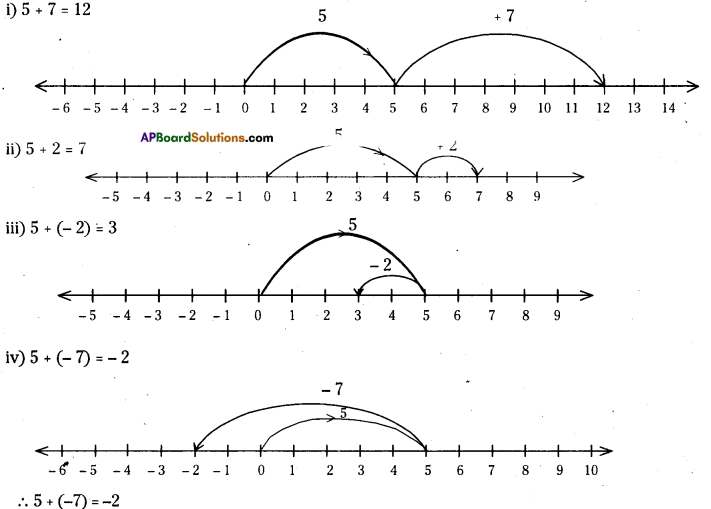AP State Syllabus AP Board 7th Class Maths Solutions Chapter 1 Integers Ex 2 Textbook Questions and Answers.

## AP State Syllabus 7th Class Maths Solutions 1st Lesson Integers Exercise 2Question 1.
Represent the following additions on a number line.
(i) 5 + 7
(ii) 5 + 2
(iii) 5 + ( – 2)
Solution:Question 2.
Compute the following.
(i) 7 + 4
(ii) 8+( – 3)
(iii) 11 + 3
(iv) 14 + ( – 6)
(v) 9 + ( – 7)
(vi) 14+( – 10)
(vii) 13 + ( – 15)
(viii) 4 + ( – 4)
(ix) 10 +( – 2)
(x) 100 +( – 80)
(xi) 225 +( – 145)
Solution:
(i) 7 + 4 = 11
(ii) 8 +( – 3) = 5
(iii) 11 + 3 = 14
(iv) 14 + ( – 6) = 8
(v) 9 + ( – 7) = 2
(vi) 14 +( – 10) = 4
(vii) 13 + ( – 15) = -2
(viii) 4 + ( – 4) = 0
(ix) 10 +( – 2) = 8
(x) 100 +( – 80) = 20
(xi) 225 +( – 145) = 80